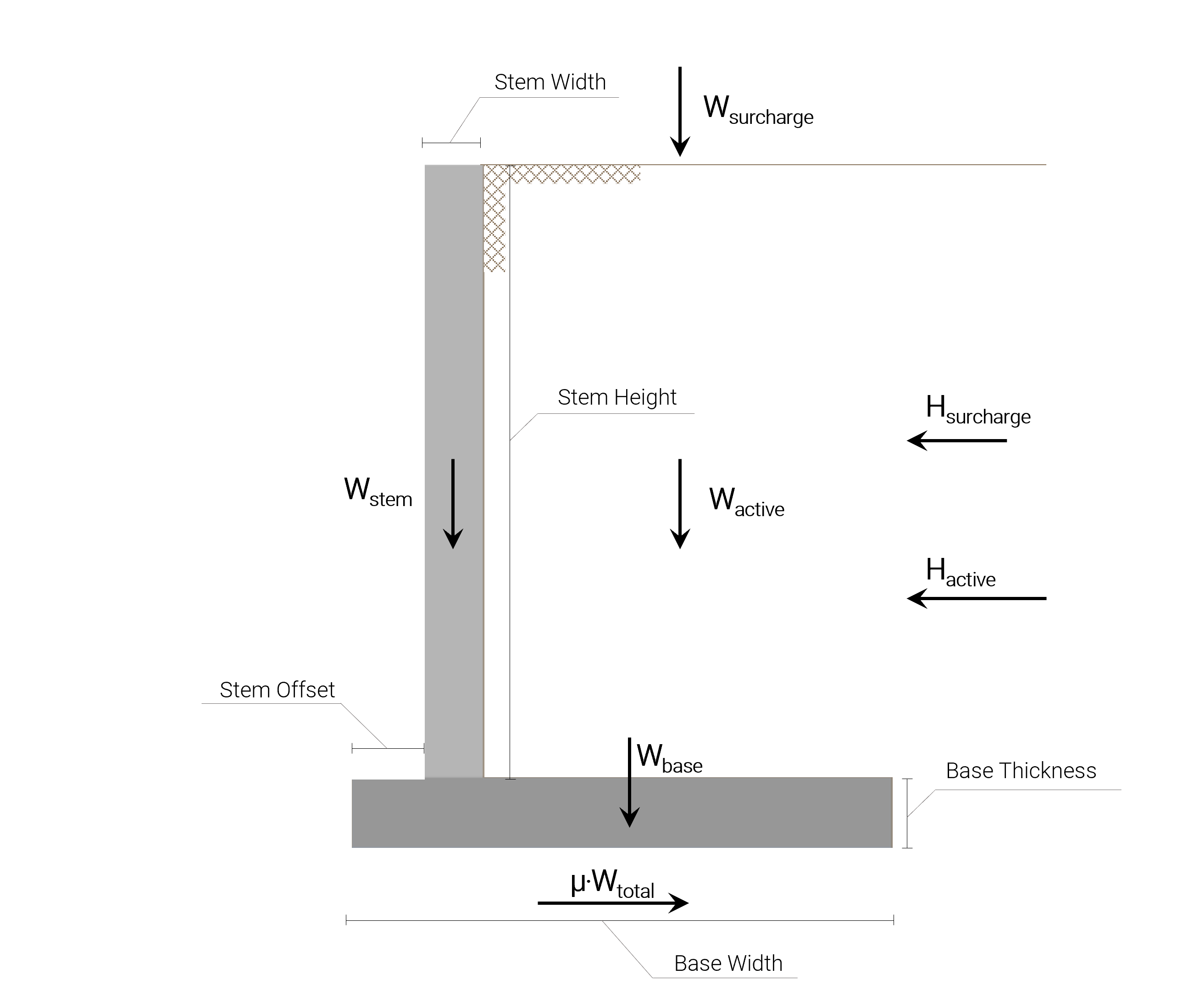SkyCiv文档

1. SkyCiv挡土墙
2. 文章和教程
3. 挡土墙滑动计算示例

# 挡土墙滑动计算示例

## How to Calculate Factor of Safety Against Sliding for Retaining Wall – 钢筋混凝土悬臂

This retaining wall sliding calculation example is a simple guide on how to calculate the Factor of Safety against sliding in a retaining wall as part of the stability checks. This sliding check is performed to ensure that the resulting force of the friction between the wall and the substructure soil is enough to prevent the Retaining Wall from sliding as a result of the horizontal loads rising from the retained soil active pressure. 基本上, the friction force that will prevent the wall from sliding is the total vertical load multiplied by the Soil-Concrete Friction Coefficient defined for the substructure soil material and the sliding force is the result of the retained soil’s lateral pressure and the pressure associated with the surcharge presence.

### 输入数据:

• 高度: 3.124 米
• 宽度: 0.305 米
• 抵消: 0.686 米

• 宽度: 2.210 米
• 厚度: 0.381 米

• 单位重量: 18.85 千牛/米3
• 摩擦角: 35 度数

• 单位重量: 18.85 千牛/米3
• 摩擦角: 35 度数
• 土-混凝土摩擦系数: 0.55
• 允许承压: 143.641 千帕

• 积极的: 3.505 米
• 被动的: 0.975 米
• 子结构: 0.792 米

All the loads associated with the Retaining Wall Sliding Calculation are shown in the following picture:### Sliding force

$$K_a = \frac{1-\没有(\伽玛_{泥,\;积极的})}{1+\没有(\伽玛_{泥,\;积极的})}$$

$$K_a = \frac{1-\没有(35º)}{1+\没有(35º)} = 0.271$$

$$H_{积极的} = frac{1}{2} \cdot \gamma_{泥,\;积极的} \点 (干_{高度} + 根据_{厚度})^{2} \cdot K_a$$

$$H_{积极的} = frac{1}{2} \点 18.85\;kN/m^3 \cdot 3.505^{2} \点 0.271$$

$$H_{积极的} = 31.377\;千牛/米$$

$$H_{泥,\;情商} = frac{附加费_{值}}{\伽玛_{泥,\;积极的}} = frac{17.237 \;千牛/米}{17.237 \;千牛/米}$$

$$H_{泥,\;情商} = 0.914 \; 米$$

$$H_{附加费} = \gamma_{泥,\;积极的} \cdot h_{泥,\;情商} \点 (干_{高度} + 根据_{厚度}) \cdot K_a$$

$$H_{附加费} =\cdot 18.85\;kN/m^3 \cdot 0.914 \; m \cdot 3.505 \; m \cdot 0.271$$

$$H_{附加费} = 16.372\;千牛/米$$

With those two loads calculated, it is now possible to calculate the sliding force by summing the two loads up:

$$\西格玛{H} = H_{积极的} + H_{附加费} = 31.377\;千牛/米 + 16.372\;千牛/米$$

$$\西格玛{H} = 47.749 \; 千牛$$

### Friction force

In order to calculate the friction force that resists the sliding force, it is necessary to evaluate the total vertical load first:

$$W_{干} = \gamma_{具体} \点 (干_{高度} \cdot 词干_{宽度} ) = 23.58 \;kN/m^3 \cdot 3.124\;m \cdot 0.305\;m$$

$$W_{干}= 22.467\;千牛/米) \(W_{以及五个节点} = \gamma_{具体} \点 (根据_{厚度} \cdot base_{宽度} ) = 23.58 \;kN/m^3 \cdot 0.381\;m \cdot 2.210\;m$$

$$W_{以及五个节点}= 18.855\;千牛/米) \(W_{积极的} = \gamma_{泥,\;积极的} \点 (干_{高度}\点 (根据_{宽度}-干_{抵消}-干_{宽度}) )$$

$$W_{积极的} = 18.85 \;kN/m^3 \cdot 3.124\;m \cdot (2.210-0.686-0.305)\;m$$

$$W_{积极的} = 71.784\;千牛/米) \(W_{附加费} = 附加费_{值} \点 ( (根据_{宽度}-干_{抵消}-干_{宽度} )$$

$$W_{附加费} = 17.237 \;kN/m \cdot (2.210-0.686-0.305)\;m$$

$$W_{附加费} = 21.012\;千牛/米) Having all those individual loads it is now possible to calculate the friction force: \( \mu \cdot \Sigma{w ^} = \mu \cdot (W_{干}+W_{以及五个节点}+W_{积极的}+W_{附加费})$$

$$\mu \cdot \Sigma{w ^} = 0.55 \点 (22.467+18.855+71.784+21.012)\;千牛$$

$$\mu \cdot \Sigma{w ^} = 0.55 \点 135.12\;千牛$$

$$\mu \cdot \Sigma{w ^} = 74.315\;千牛$$

### Factor of Safety against sliding

$$FS = \frac{\mu \cdot \Sigma{w ^}}{\西格玛{H}}$$

$$FS = \frac{74.315\;千牛}{47.749 \; 千牛}= 1.556 \葛 1.5$$ 通过!

## 挡土墙计算器

SkyCiv offers a free Retaining Wall Calculator that will check sliding in retaining wall and perform a stability analysis on your retaining walls. 付费版本还显示完整的计算, 所以你可以一步一步看到, 挡土墙抗倾覆稳定性如何计算, 滑动轴承!ng (民用)